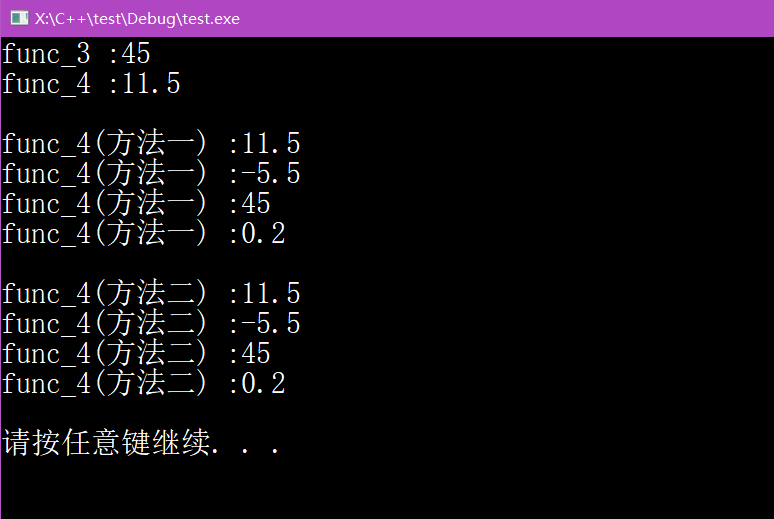# C++函数指针和回调函数

bool lengthCompare(const string &, const string &);


bool （*pf）(const string &, const string &);		//pf是一个函数指针


pf = lengthCompare;

pf = &lengthCompare;


typedef定义函数指针类型

typedef bool func(const string &, const string &);
typedef bool (*myFunc) (const string &, const string &);


myFunc p_func = nullptr;


void useBigger(const string &, const string &, lengthCompare);


using F = int (int*, int);			//F是函数类型
using PF = int (*) (int*, int);	//PF是指针类型


#include <iostream>
#include <cstdlib>

using namespace std;

typedef float (*p_func)(int, int, float);

//回调函数
float func_1(int a, int b, float c)
{
return a*b*c;
}

//回调函数
float func_2(float a, float b, float c)
{
return a+b+c;
}

float func_3(int a, int b, float c, p_func p)
{
return (*p)(a,b,c);		//等价于 return *p(a,b,c);
}

float func_4(int a, int b, float c, float (*p_ff)(float, float, float))
{
return (*p_ff)(a,b,c);	//等价于 return *p_ff(a,b,c);
}

//下面4个函数都是回调函数
float func_add(float a, float b, float c)
{
return a+b+c;
}
float func_sub(float a, float b, float c)
{
return a-b-c;
}
float func_mul(float a, float b, float c)
{
return a*b*c;
}
float func_div(float a, float b, float c)
{
return a/b/c;
}

int main()
{
cout << "func_3 :" << func_3(3, 6, 2.5, func_1) << endl;
cout << "func_4 :" << func_4(3, 6, 2.5, func_2) << endl << endl;

//方法一：定义函数指针数组
float(*f_p_a)(float, float, float);
f_p_a = func_add;
f_p_a = func_sub;
f_p_a = func_mul;
f_p_a = func_div;

cout << "func_4(方法一) :" << func_4(3, 6, 2.5, f_p_a) << endl;
cout << "func_4(方法一) :" << func_4(3, 6, 2.5, f_p_a) << endl;
cout << "func_4(方法一) :" << func_4(3, 6, 2.5, f_p_a) << endl;
cout << "func_4(方法一) :" << func_4(3, 6, 2.5, f_p_a) << endl << endl;

//方法二：使用typedef
typedef float (*f_p_a_type)(float, float, float);
f_p_a_type ff_pp_aa = {func_add, func_sub, func_mul, func_div};

cout << "func_4(方法二) :" << func_4(3, 6, 2.5, ff_pp_aa) << endl;
cout << "func_4(方法二) :" << func_4(3, 6, 2.5, ff_pp_aa) << endl;
cout << "func_4(方法二) :" << func_4(3, 6, 2.5, ff_pp_aa) << endl;
cout << "func_4(方法二) :" << func_4(3, 6, 2.5, ff_pp_aa) << endl << endl;

system("pause");
return 0;
}09-10588
06-216万+
03-29
11-111万+
03-27242
03-16276
07-12180
03-29190
09-042783
06-164772
10-05702
03-282650
04-13753
01-08464
03-25532
05-203148
09-163万+
©️2020 CSDN 皮肤主题: 书香水墨 设计师:CSDN官方博客